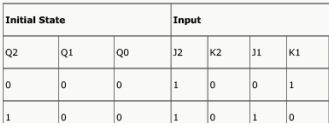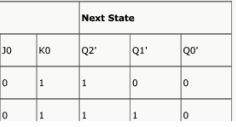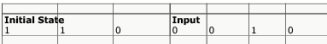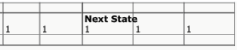Courses

# Circuit Outputs MCQ Quiz - 1

## 30 Questions MCQ Test RRB JE for Computer Science Engineering | Circuit Outputs MCQ Quiz - 1

Description
This mock test of Circuit Outputs MCQ Quiz - 1 for Computer Science Engineering (CSE) helps you for every Computer Science Engineering (CSE) entrance exam. This contains 30 Multiple Choice Questions for Computer Science Engineering (CSE) Circuit Outputs MCQ Quiz - 1 (mcq) to study with solutions a complete question bank. The solved questions answers in this Circuit Outputs MCQ Quiz - 1 quiz give you a good mix of easy questions and tough questions. Computer Science Engineering (CSE) students definitely take this Circuit Outputs MCQ Quiz - 1 exercise for a better result in the exam. You can find other Circuit Outputs MCQ Quiz - 1 extra questions, long questions & short questions for Computer Science Engineering (CSE) on EduRev as well by searching above.
QUESTION: 1

### How many types of sequential circuits are?

Solution:

There are two type of sequential circuits viz., (i) synchronous or clocked and (ii) asynchronous or unclocked. Synchronous Sequential Circuits are triggered in the presence of a clock signal, whereas, Asynchronous Sequential Circuits function in the absence of a clock signal.

QUESTION: 2

Solution:
QUESTION: 3

### Multiple choices can be correct. Mark all of them. For the initial state of 000, the function performed by the arrangement of the J-K flip-flops in figure is: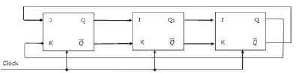Solution:

circuit behaves as shift register and mod6 counter
clock cycle    output
1                    100
2                    110
3                    111
4                    011
5                    001
6                    000

EDIT- This is Johnson counter which is application of Shift Register. And Johnson counter is  mod 2N  counter.

QUESTION: 4

The logic expression for the output of the circuit shown in figure below is: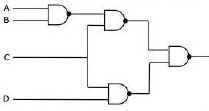Solution:

(((AB)' C)' (CD)')' = ((AB)'C) + CD) = (A' + B')C + CD = A'C + B'C + CD

QUESTION: 5

Consider the circuit in Fig.2.21 which has a four bit binary number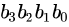as input and a five bit binary number,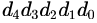as output.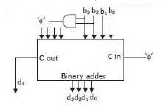Solution:

when b3b2 is 11 restore circuit to 0000..
it counts from 0 to 11 i.e. Radix 12 no ..
circuit used for  binary to radix 12 conversion..

QUESTION: 6

Consider the circuit in figure. f implements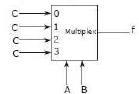Solution:

Options for this question seem wrong, its expression f will come to A'B'C + A'BC + AB'C + ABC, that further can be minimized to C.

QUESTION: 7

Consider a logic circuit shown in figure below. The functions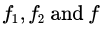(in canonical sum of products form in decimal notation) are :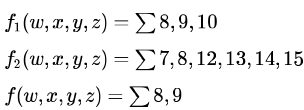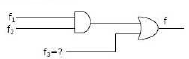The function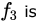Solution: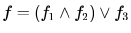Since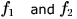are in canonical sum of products form,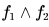will only contain their common terms- that is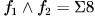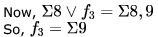QUESTION: 8

Consider the circuit shown below. In a certain steady state, the line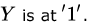. What are the possible values of  and in this state?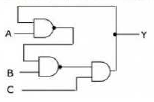Solution:

The figure is not clear- I assume there is a NOT gate just before taking Y making the final AND gate a NAND gate.
We have a steady state- meaning output is not changing. Y is 1 and remains 1 in the next state(s). So, we can write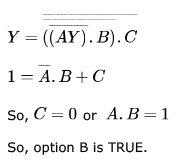QUESTION: 9

The following arrangement of master-slave flip flops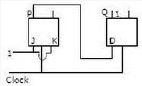has the initial state of P, Q as 0, 1 (respectively). After a clock cycle the output state P, Q is (respectively),

Solution:

Here clocks are applied to both flip flops simultaneously
When 11 is applied to jk flip flop it toggles the value of P so op at  P will be 1
Input to D flip flop will be 0( initial value of P)  so op at Q will be 0
So ans is a

QUESTION: 10

Consider the following circuit with initial state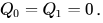The D Flip-flops are positive edged triggered and have set up times 20 nanosecond and hold times 0.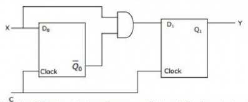Consider the following timing diagrams of X and C. The clock period of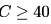nanosecond. Which one is the correct plot of Y?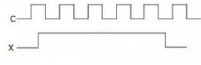Solution:

Given clock is + edge triggered.
See the first positive edge. X is 0, and hence output is 0. Q0 is 1 and Q0' is 0.
Second + edge, X is 1 and Q0' is also one. So, output is 1. (When second positive edge of the clock arrives, Q0' would surely be 1 because the set up time of flip flop is given as 20 ns and the clock period is >= 40 ns)
Third + edge, X is 1 and Q0' is 0, So, output is 0. (Q0' becomes 0 before the 3rd positive edge, but output Y won't change as the flip flop is positive edge triggered)
Now, output never changes back to 1 as Q0' is always 0 and when Q0' finally becomes 1, X is 0.
Set up time and hold times are given just to ensure that edge triggering work properly.

QUESTION: 11

Consider the following multiplexer where 10, 11, 12, 13 are four data input lines selected by two address line combinations A1A0=00,01,10,11 respectively and f is the output of the multiplexor. EN is the Enable input.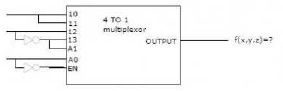The function f(x,y,z) implemented by the above circuit is

Solution:

As X connected to I0 & I1 .Y connected to I2, Y' connected to I3 & A1 , Z connected to A0 and Z' connected to ENABLE (EN).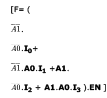F = (XYZ' +XYZ + Y'ZY + ZY')Z'
= (XYZ' +XYZ + ZY')Z'
=XYZ'

QUESTION: 12

Consider the partial implementation of a 2-bit counter using T flip-flops following the sequence 0-2-3-1-0, as shown below.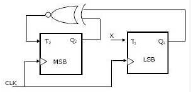Solution:

sequence is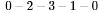From the given sequence, we have state table as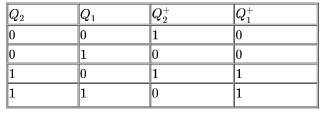Now we have present state and next state, use excitation table of T flip-flop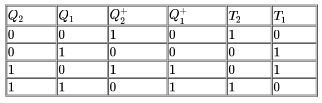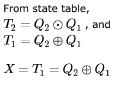QUESTION: 13

Consider the following circuit.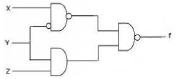Which one of the following is TRUE?

Solution:

The expression will be
f = [(x.y')'.(y.z)]'=[(x'+y).(y.z)] =[x'.y.z+y.z]'=[(x'+1).(y.z)]'=[1.(y.z)]'=[y.z ]'=y'+z'
The final expression only contains y and z,
Therefore, answer will be (a) f is Independent of x

QUESTION: 14

Consider the following circuit involving a positive edge triggered D FF.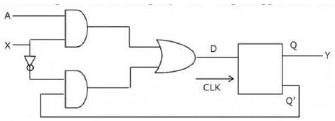Consider the following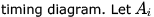. represents the logic level on the line a in the i-th clock period.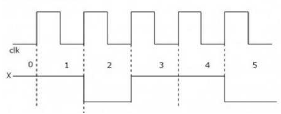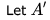represent the compliment of A. The correct output sequence on A over the clock periods 1 through  is:

Solution:

D = AX + X'Q'
Y = D
Ai represent the logic level on the line A at the i-th clock period. If we see the timing diagram carefully, we can see that during the rising edge, the output Y is determined by the X value just before that rising edge. i.e., during the rising edge say for clk2, X value that determines the output is 1 and not 0 (because it takes some propagation delay for the 0 to reach the flip flop). Similarly, the A output that determines the output for clk i, is Ai-1
For clk1, X is 1, so, D = A = A0
For clk2, X is 1, so D = A = A1
For clk 3, X is 0, so D = Q2' = A1'
For clk4, X is 1, so D = A = A3
For clk5, X is 1, so D = A = A4

QUESTION: 15

Consider the following circuit: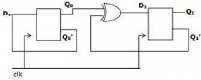The flip-flops are positive edge triggered D FFs. Each state is designated as a two-bit string Q0Q1. Let the initial state be 00. The state transition sequence is

Solution:

Clearly Q0 alternates in every clk cycle as Q0' is fed as input and it is D flipflop.

Q1 becomes 1 if its prev value and current Q0 differs (EXOR).
So, the sequence of transitions will be 00 -> 11 -> 01 -> 10 -> 00 (D) choice.

QUESTION: 16

A two-way switch has three terminals a, b and c. In ON position (logic value 1), a is connected to b, and in OFF position, a is connected to c. Two of these two-way switches S1 and S2 are connected to a bulb as shown below.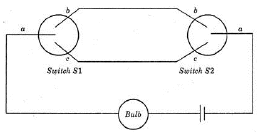Which of the following expressions, if true, will always result in the lighting of the bulb ?

Solution:

If it's looked carefully, bulb will be on when both switch s1 and s2 are in same state, either off or on. that is exnor operation
S1 S2  Bulb
0   0    On
0   1    Off
1   0    Off
1   1   On
it's Ex-NOR operation hence (C) is the correct option.

QUESTION: 17

Which of the following input sequences will always generate a 1 at the output z at the end of the third cycle?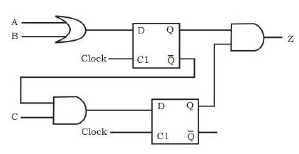Solution: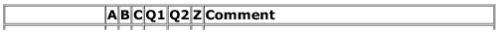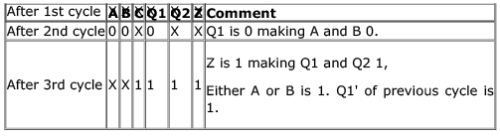The filling is done in reverse order. Here, none of the options matches. So, something wrong somewhere.

QUESTION: 18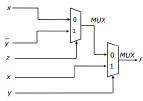Consider the circuit above. Which one of the following options correctly represents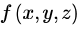Solution:

if you solve this you will get XY' + Y'Z + XY (this can be simplified to X + Y'Z) with min terms as (1,4,5,6,7)
and option A has the same min terms
so option A is equivalent to XY' + Y'Z + XY

Result of MUX (first one), is,say f1, = xz'+ y'z
Result of MUX(second one , f= f1y' +xy
=(xz'+y'z)y'+xy  = xy'z' +y'z +xy =x(y'z' +y) +y'z  = x(y'+y)(z'+y) +y'z   =xz'+xy+y'z .
Option A.
Note:
1. f =I0S' +I1S ,for 2:1 MUX , where I0 and I1 are inputs , S is select line 2. Distributive property , A+BC = (A+B)(A+C)
3. A+A' =1

QUESTION: 19

Consider the circuit in the diagram. The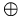operator represents Ex-OR. The D flip-flops are initialized to zeroes (cleared).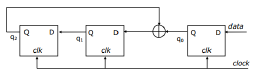The following data: 100110000 is supplied to the “data” terminal in nine clock cycles. After that the values of q2q1q0 are:

Solution: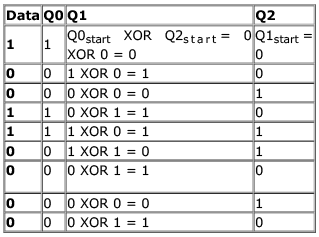QUESTION: 20

The control signal functions of a 4-bit binary counter are given below (where X is “don’t care”):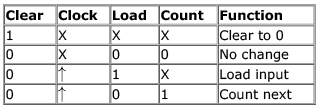The counter is connected as follows: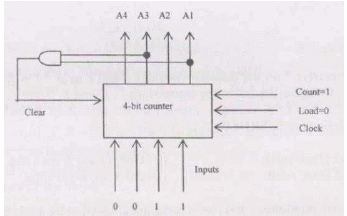Assume that the counter and gate delays are negligible. If the counter starts at 0, then it cycles through the following sequence:

Solution:

whenever  A4 A3 A2 A1 =0 1 0 1 then clear line will be enabled as A3 and A1 set.
given table says that whenever clear control signal set , it clears to 0 0 0 0 , before the current clock cycle completes.
so 5 is cleared to 0 in the same clock cycle.
so counter sequence is 0, 1 , 2, 3, 4

QUESTION: 21

The following expression was to be realized using 2-input AND and OR gates. However, during the fabrication all 2-input AND gates were mistakenly substituted by 2-input NAND gates. (a.b).c + (a'.c).d + (b.c).d + a. d

What is the function finally realized ?

Solution:

the final answer will come as --
a'+c'+d'+a'c+ab+bc
= a'(c+1)+c'+d'+ab+bc
=a'+c'+d'+ab+bc
=(a'+a)(a'+b)+(c'+c)(c'+b)+d' =a'+b+c'+b+d'
=a'+b+c'+d'

QUESTION: 22

The line T in the following figure is permanently connected to the ground.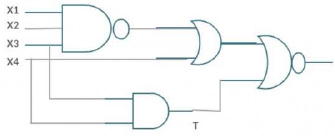Which of the following inputs (X1 X2 X3 X4) will detect the fault ?

Solution:

To detect the fault, we should get an unexpected output. The final gate here is a NOR gate which produces output 0 if either of its input is 1 and else 1. i.e., the output will be 0 for inputs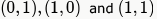and  and output will be 1 for (0,0).
By grounding T is at 0. So, we can ignore the inputs (1,0) and (0,0) to the final NOR gate as they won't be detecting faults. Now, expected (1,1) input will become (1,0) due to grounding of T but produces same output 0 as for (1,1).
Hence this also cannot detect the defect. So, to detect the defect, the input to the final gate must be (1,1) which is expected to produce a 0 but will produce a 1 due to grounding of T.

Now, for (0,1) input for the final gate, we must have,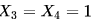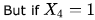, the OR gate makes 1 output and we won't get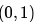input for the final gate. This means, no input sequence can detect the fault of the circuit.
Alternatively, we can write equation for the circuit as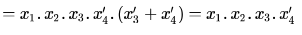For the faulty circuit output will be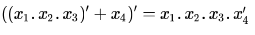So, there is no effect of T being grounded here. Answer is D option.

QUESTION: 23

What is the final value stored in the linear feedback shift register if the input is 101101?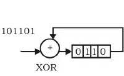Solution:

The four bit register contains: 1011, 1101, 0110, 1011, 1101, 0110 after each shift.

QUESTION: 24

What Boolean function does the circuit below realize?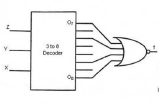Solution:

F = (x'z' + xz)' = xz' + x'z

QUESTION: 25

What is the boolean expression for the output f of the combinational logic circuit of NOR gates given below?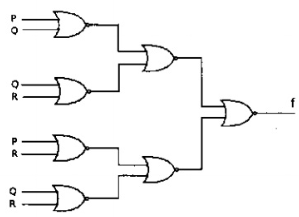Solution: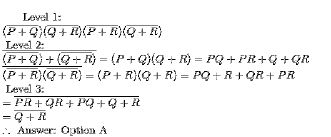QUESTION: 26

In the sequential circuit shown below, if the initial value of the output Q1Q0 is 00. What are the next four values of Q1Q0 ?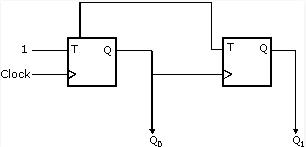Solution:

Initially Q0Q1 = 00
after first clock signal Q0 will be toggled and change to 1. It will activate clock of second FF in sequence and toggle that one as well.
Hence next output Q0Q1=11
Similarly next few sequences for Q0Q1 will be 01, 00
Hence answer sequence Q1Q0 will be 11,10,01,00

QUESTION: 27

The Boolean expression of the output f of the multiplexer shown below is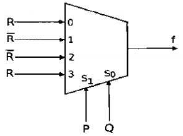Solution: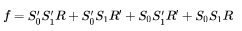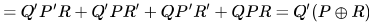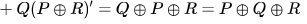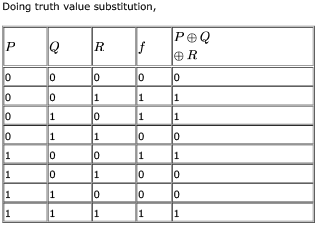QUESTION: 28

Consider the following circuit involving three D-type flip-flops used in a certain type of counter configuration.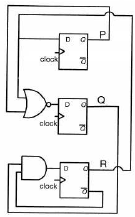If at some instance prior to the occurrence of the clock edge,
P,Q and R have a value 0, 1 and 0 respectively, what shall be the value of PQR after the clock edge

Solution: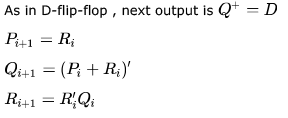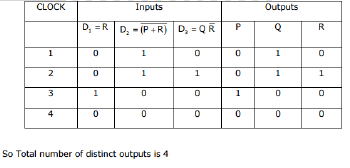QUESTION: 29

Consider the following circuit involving three D-type flip-flops used in a certain type of counter configuration.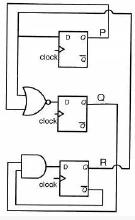If all the flip-flops were reset to 0 at power on, what is the total number of distinct outputs (states) represented by PQR generated by the counter?

Solution: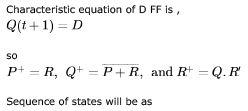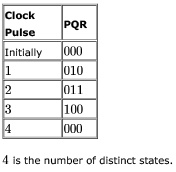QUESTION: 30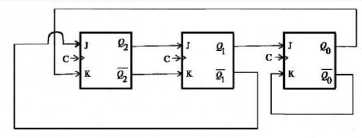The above synchronous sequential circuit built using JK flip-flops is initialized with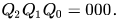The state sequence for this circuit for the next 3 clock cycles is

Solution: